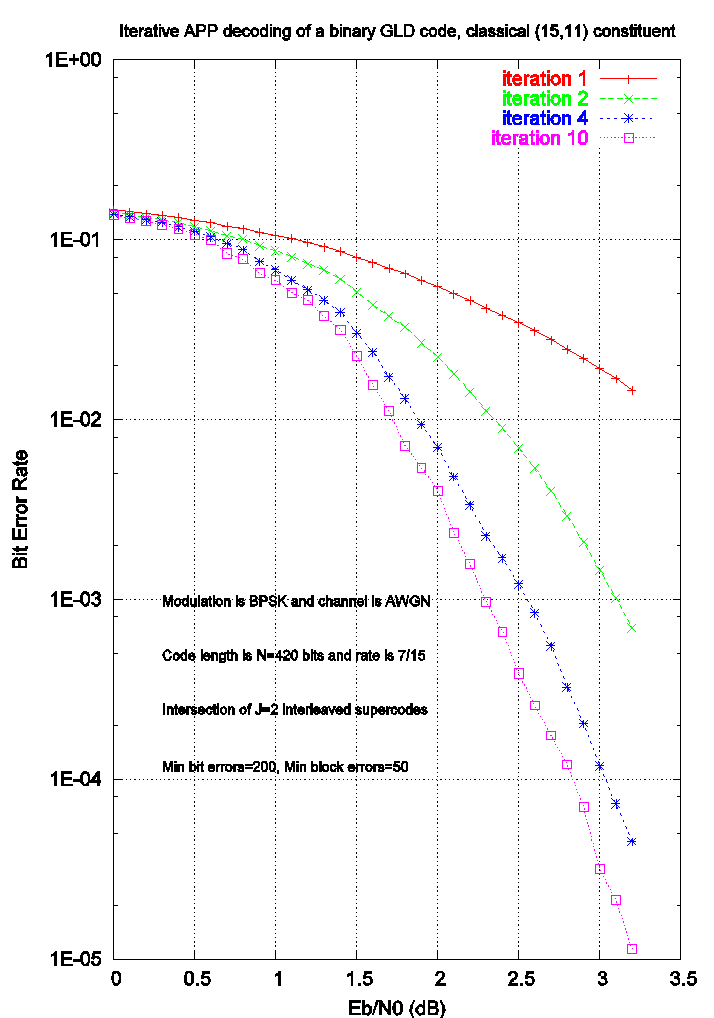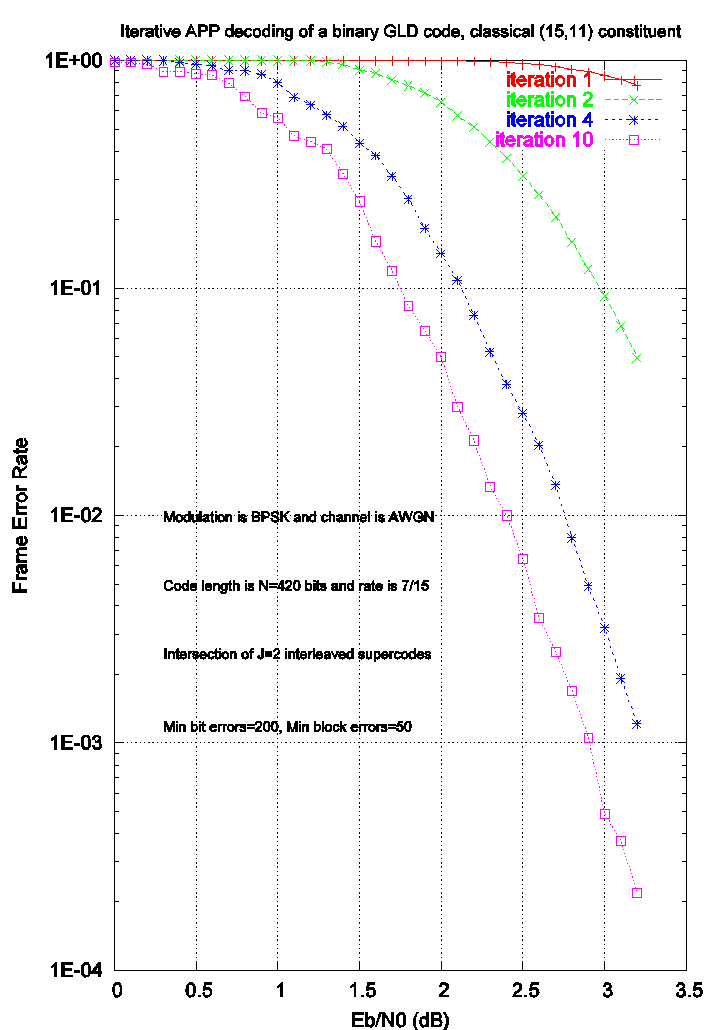Generalized Low Density (Tanner) Codes

Last updated on April 2, 2004

 You find here a computer simulation and a database for a binary GLD code built from the (15,11) Hamming code. The rate of the GLD code is 7/15=0.4666 and its length is 420 bits. The degree of binary digits is J=2. All files given below have been generated under RedHat Linux version 9 via LIBCOM 3. Please, text files (.txt) and data files (.dat) should be read using Word or Wordpad under Windows, or any text editor under Unix/Linux.► The GLD parity-check matrix (non-systematic form) of size 224x420 in file Hmatrix_GLD_N=420_K=196_n=15_k=11_inter=3_seed=295.txt

► The GLD parity-check matrix (systematic form) of size 224x420 in file Hmatrix_sys_GLD_N=420_K=196_n=15_k=11_inter=3_seed=295.txt

► The 62 column permutations linking the above matrices in file Perms_GLD_N=420_K=196_n=15_k=11_inter=3_seed=295.txt

► Bit error rate performance after 20 half-iterations versus Eb/N0 in file bch_gld_N=420_K=196_n=15_k=11_inter=3_seed=295_ber.dat

► Figure summarizing the BER performance (landscape) in file bch_gld_N=420_K=196_n=15_k=11_inter=3_seed=295_ber_land.eps

► Figure summarizing the BER performance (portrait) bch_gld_N=420_K=196_n=15_k=11_inter=3_seed=295_ber_port.eps

► Frame error rate performance after 20 half-iterations versus Eb/N0 in file bch_gld_N=420_K=196_n=15_k=11_inter=3_seed=295_fer.dat

► Figure summarizing the FER performance (landscape) in file bch_gld_N=420_K=196_n=15_k=11_inter=3_seed=295_fer_land.eps

► Figure summarizing the FER performance (portrait) in file bch_gld_N=420_K=196_n=15_k=11_inter=3_seed=295_fer_port.eps

Biography Zone

A low complexity FEC scheme based on the intersection of interleaved block codes,

by O. Pothier, L. Brunel and J.J. Boutros, IEEE Vehicular Technology Conference, vol. 1, pp. 274-278, Houston, USA, May 1999.

Generalized low density (Tanner) codes,

by J.J. Boutros, O. Pothier and G. Zémor, IEEE International Conference on Communications, vol. 1, pp. 441-445, Vancouver, Canada, June 1999.

Image Zone